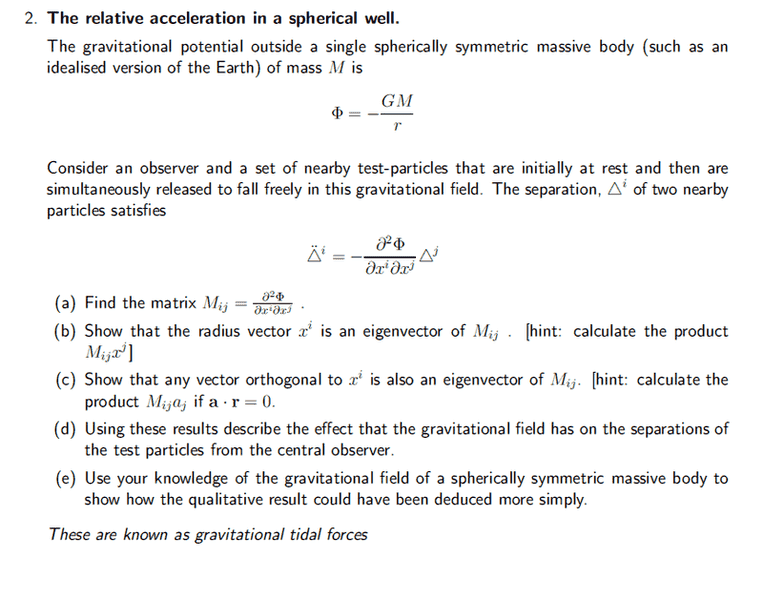# General Relativity - Index Notation

• unscientific

## Homework Statement

(a) Find matrix element ##M_{ij}##
(b) Show that ##x^j## is an eigenvector of ##M_{ij}##
(c) Show any vector orthogonal to ##x^j## is also an eigenvector of ##M_{ij}#### The Attempt at a Solution

Part(a)
[/B]
$$\frac{\partial^2 \Phi}{\partial x^i x^j} = -\frac{2GM_i}{(x^i)^3} \delta_{ij}$$

Part(b)

$$M_{ij}x_j = \frac{-2GM_i}{(x^i)^2}$$

How do I show it is an eigenvector? It is clearly not..

Hi. What you obtain in (a) is wrong: the double derivative should give you two terms, the denominator should be a power of r and the index on M is probably a typo...

Goddar said:
Hi. What you obtain in (a) is wrong: the double derivative should give you two terms, the denominator should be a power of r and the index on M is probably a typo...
Sorry I don't quite follow..

Can you detail your calculation of Mij so maybe i can tell you what goes wrong?

Goddar said:
Can you detail your calculation of Mij so maybe i can tell you what goes wrong?

Part(a)
$$M_{ij} = \frac{\partial^2}{\partial x^j x^i} GM(x^i x^i)^{-\frac{1}{2}} = -GM \frac{\partial}{\partial x^j} x^i (x^i x^i)^{-\frac{3}{2}} = 3GMx^i x^j (x^i x^i)^{-\frac{5}{2}} \delta_{ij}$$

Part (b)

$$M_{ij} x^j = 3GMx^i (x^j)^2 (x^i x^i)^{-\frac{5}{2}} \delta_{ij} = 3GM (x^i x^i)^{-2}$$

Ok. First i would recommend you actually expand r in terms of x,y and z instead of using summation convention: it will be less confusing and you'll see what's going on more clearly.
But if you want to use it, you have to pick a different index for your denominator (not i or j)!
Then you will see that in the second derivative you wrongfully assigned a Kronecker delta to 3GMxixj/r5,
while you're forgetting a term for the case where i = j (so that this second term does come with a Kronecker delta!).
If you do all that you'll see how part (b) comes out as expected.

Goddar said:
Ok. First i would recommend you actually expand r in terms of x,y and z instead of using summation convention: it will be less confusing and you'll see what's going on more clearly.
But if you want to use it, you have to pick a different index for your denominator (not i or j)!
Then you will see that in the second derivative you wrongfully assigned a Kronecker delta to 3GMxixj/r5,
while you're forgetting a term for the case where i = j (so that this second term does come with a Kronecker delta!).
If you do all that you'll see how part (b) comes out as expected.

Oh I think I got it now. I should have used a dummy index for ##r##.

Part(a)

$$M_{ij} = \frac{\partial^2}{\partial x^j x^i} GM(x^l x^l)^{-\frac{1}{2}} = -GM \frac{\partial}{\partial x^j} x^i (x^l x^l)^{-\frac{3}{2}} = -GM \left[ \frac{\partial}{\partial x^i} x^i (x^l x^l)^{-\frac{3}{2}} + \frac{\partial}{\partial x^{j \neq i}} x^i (x^l x^l)^{-\frac{3}{2}} \right]$$

$$M_{ij} = -GM \left[ (x^l x^l)^{-\frac{3}{2}} -3 (x^i)^2 (x^l x^l)^{-\frac{5}{2}} -3 x^i x^j (x^l x^l)^{-\frac{5}{2}} \delta_{ij} \right]$$

Part (b)

$$M_{ij} x^j = GM \left[ x^j (x^l x^l)^{-\frac{3}{2}} -3 x^j (x^i)^2 (x^l x^l)^{-\frac{5}{2}} -3 x^i (x^j)^2 (x^l x^l)^{-\frac{5}{2}} \delta_{ij} \right]$$

Using the kronecker delta,
$$M_{ij} x^j = GM (x^l x^l)^{-\frac{3}{2}} x^j$$

Last edited:
You're getting there but there's still a mistake in your second derivative (again it would probably appear more clearly if you used explicit expressions of x,y,z):
1 - you obtain 3 terms while there should be only two because the xj in the denominator gets a derivative whether or not j = i
2 - the first term you obtain should be the one carrying a Kronecker delta since it is the one corresponding solely to the case where j = i

Goddar said:
You're getting there but there's still a mistake in your second derivative (again it would probably appear more clearly if you used explicit expressions of x,y,z):
1 - you obtain 3 terms while there should be only two because the xj in the denominator gets a derivative whether or not j = i
2 - the first term you obtain should be the one carrying a Kronecker delta since it is the one corresponding solely to the case where j = i

You're right, I'm complicating things. Here's what it should be:

Part(a)

$$M_{ij} = \frac{\partial^2}{\partial x^j x^i} GM(x^l x^l)^{-\frac{1}{2}} = -GM \frac{\partial}{\partial x^j} x^i (x^l x^l)^{-\frac{3}{2}} = -GM \left[ \delta_{ij}(x^l x^l)^{-\frac{3}{2}} -3 x^j x^i (x^l x^l)^{-\frac{5}{2}}\right]$$

Part (b)

$$M_{ij} x^j = -GM \left[ \delta_{ij} x^j (x^l x^l)^{-\frac{3}{2}} -3 (x^j x^j) x^i (x^l x^l)^{-\frac{5}{2}} \right] = -GM \left[ \delta_{ij} x^j (x^l x^l)^{-\frac{3}{2}} -3 x^i (x^l x^l)^{-\frac{3}{2}} \right] = 2 GM (x^l x^l)^{-\frac{3}{2}} x^j$$

Last edited:
Yep. Now you can express again the denominator as r-3, so it's obvious that xj is an eigenvector...

Goddar said:
Yep. Now you can express again the denominator as r-3, so it's obvious that xj is an eigenvector...
Thanks man! I will try out the remaining parts and update it here